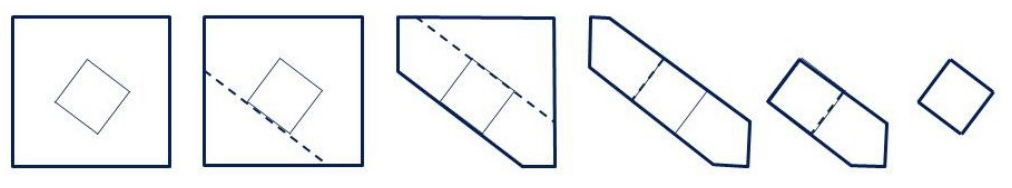시간 제한 메모리 제한 제출 정답 맞은 사람 정답 비율
2 초 512 MB 13 9 9 69.231%

## 문제

You are given two convex polygons A and B. It is guaranteed that B is strictly contained inside of A.

You would like to make a sequence of cuts to cut out B from A. To do this, you draw a straight line completely through A that is incident to one of the edges of B, which separates A into two pieces. You cut along this line and discard the piece that doesn’t contain B. You repeat this until the piece that you have left is exactly B.The cost of making a cut is equal to the length of the cut (i.e. the length of the line through the remainder of A). Given A and B, find the minimum cost needed to cut B out.

## 입력

Each input will consist of a single test case. Note that your program may be run multiple times on different inputs. Each test case will begin with a line containing a single integer a (3 ≤ a ≤ 200), which is the number of points in polygon A. Each of the next a lines will contain two integers x and y (−106 ≤ x, y ≤ 106), which are the vertices of polygon A, in clockwise order. It is guaranteed that polygon A will be convex.

The next line will contain a single integer b (3 ≤ b ≤ 200), which is the number of points in polygon B. Each of the next b lines will contain two integers x and y (−106 < x, y < 106), which are the vertices of polygon B, in clockwise order. It is guaranteed that polygon B will be convex. It is also guaranteed that polygon B will reside entirely within the interior of polygon A.

No three points, within a polygon or across polygons, will be collinear.

## 출력

Output a single floating point number, which is the minimum cost to cut B out of A. To be considered correct, this number must be within a relative error of 10−6 of the judges’ answer.

## 예제 입력 1

4
0 0
0 14
15 14
15 0
4
8 3
4 6
7 10
11 7


## 예제 출력 1

40.0000000000


## 예제 입력 2

4
-100 -100
-100 100
100 100
100 -100
8
-1 -2
-2 -1
-2 1
-1 2
1 2
2 1
2 -1
1 -2


## 예제 출력 2

322.1421356237